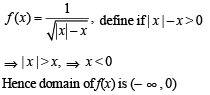# MCQs Of Functions, Past Year Questions JEE Mains, Class 12, Maths

## 17 Questions MCQ Test Maths 35 Years JEE Mains & Advance Past year Papers Class 12 | MCQs Of Functions, Past Year Questions JEE Mains, Class 12, Maths

Description
This mock test of MCQs Of Functions, Past Year Questions JEE Mains, Class 12, Maths for Class 12 helps you for every Class 12 entrance exam. This contains 17 Multiple Choice Questions for Class 12 MCQs Of Functions, Past Year Questions JEE Mains, Class 12, Maths (mcq) to study with solutions a complete question bank. The solved questions answers in this MCQs Of Functions, Past Year Questions JEE Mains, Class 12, Maths quiz give you a good mix of easy questions and tough questions. Class 12 students definitely take this MCQs Of Functions, Past Year Questions JEE Mains, Class 12, Maths exercise for a better result in the exam. You can find other MCQs Of Functions, Past Year Questions JEE Mains, Class 12, Maths extra questions, long questions & short questions for Class 12 on EduRev as well by searching above.
QUESTION: 1

### The domain of sin-1 [log3 (x/3)] is

Solution:

Domain of sin-1x is [-1,1]

Therefore, -1 ≤ log3(x/3) ≤ 1

3-1 ≤ x/3 ≤ 3

1 ≤ x ≤ 9

Therefore, the domain of sin-1[log3(x/3)] is [1,9]

QUESTION: 2

### The function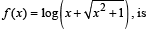Solution: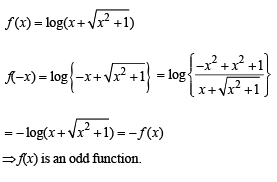QUESTION: 3

### Domain of definiti on of the function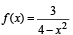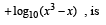Solution: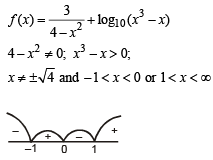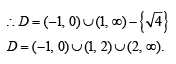QUESTION: 4

If f : R → R satisfies f (x + y) = f ( x) + f (y) , for all  x,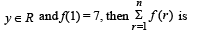Solution: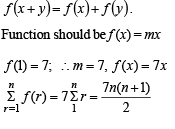QUESTION: 5

A function f from the set of  natural numbers to integers defined by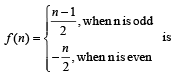Solution:

We have f: N →I
If x and y are two even natural numbers,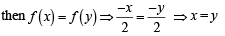Again if x and y are two odd natural numbers then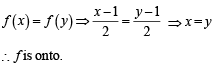Also each negative integer is an image of even natural number and each positive integer is an image of odd natural number.

∴ f is onto.
Hence f is one one and onto both.

QUESTION: 6

The range of the function f (x) = 7- x Px -3 is

Solution: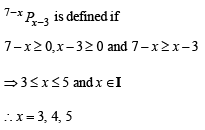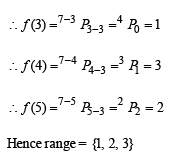QUESTION: 7

If f : R → S, defined by

f (x) = sin x - √3 cosx+ 1, is onto, then the interval of S is

Solution:

f (x) is onto ∴ S = range of f (x)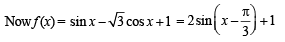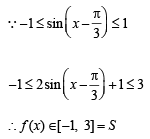QUESTION: 8

The graph of the function y = f(x) is symmetrical about the line x = 2, then

Solution:

Let us consider a graph symm. with respect to line x = 2 as shown in the figure.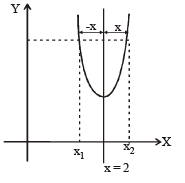From the figure

f (x1) = f (x2), where x1 = 2-x and x2 = 2+x
∴ f (2 - x) = f (2+x)

QUESTION: 9

The domain of the function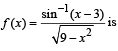Solution: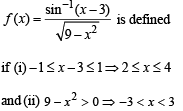Taking common solution of  (i) and (ii), we get 2 < x < 3  ∴ Domain = [2, 3)

QUESTION: 10

L et f : (– 1, 1) → B , be a function defined by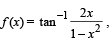then f is both one - one and onto when B is the interval

Solution: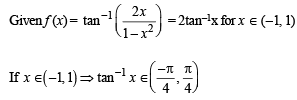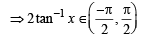Clearly, range of f (x)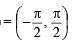For f to be onto, codomain = range
∴ Co-domain of function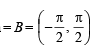QUESTION: 11

A function is matched below against an interval where it is supposed to be increasing. Which of the following pairs is incorrectly matched?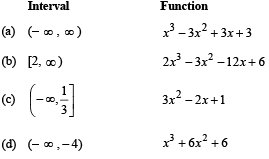Solution:

Clearly function f (x) = 3x2 - 2x+1 is increasing when
f ' (x) = 6x – 2 > 0 ⇒ x ∈[1 / 3,∞)

∴ f (x) is incorrectly matched with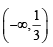QUESTION: 12

A real valued function f(x) satisfies the functional equation f(x – y) = f(x) f(y) – f(a – x) f(a + y) where a is a given constant and f(0) = 1, f(2a – x) is equal to

Solution:

f(2a – x) = f (a – (x – a))
= f(a) f(x – a) – f(0) f(x) = f(a) f(x –a) – f(x)
= – f(x)
[∴ x = 0, y = 0, f(0) = f2(0)- f2(a)
⇒ f2(a) = 0
⇒ f(a) = 0]
⇒ f(2a - x) = - f(x)

QUESTION: 13

The largest interval lying in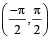for which the function,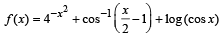, is defined, is

Solution: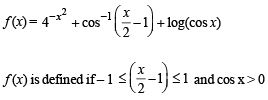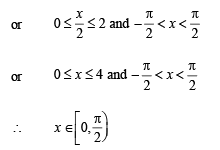QUESTION: 14

Let f: N→Y be a function defined as f(x) = 4x + 3 where Y = {y ∈ N : y = 4x + 3 for some x ∈ N}.

Show that f is invertible and its inverse is

Solution:

Clearly f is one one and onto, so invertible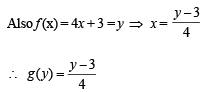QUESTION: 15

Let f(x) = ( x + 1)2 – 1,x > –1

Statement -1 : The set {x : f(x) = f –1(x) = {0, –1}

Statement-2 : f is a bijection.

Solution:

Given that f (x)  = (x + 1)2 –1,  x > –1 Clearly Df = [–, ∞) but co-demain is not given.
Therefore  f (x) need not be necessarily onto.
But if f (x) is onto then as f (x) is one one also, (x + 1) being something +ve, f–1(x) will exist where (x + 1)2 –1 = y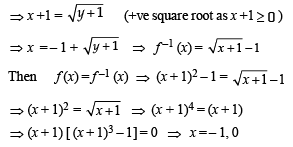∴ The statement-1 is correct but statement-2 is false.

QUESTION: 16

For real x, let f (x) = x3 + 5x + 1, then

Solution:

Given that f (x) = x3 + 5x + 1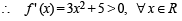⇒ f (x) is strictly increasing on R
⇒ f (x) is one one
∴ Being a polynomial f (x) is cont. and inc.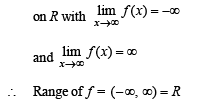Hence f is onto also. So, f is one one and onto R.

QUESTION: 17

The domain of the function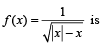Solution: